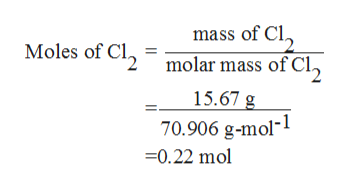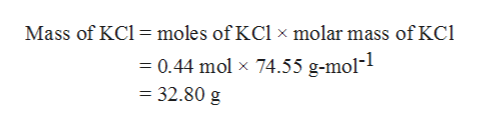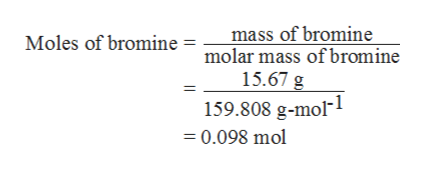# For each of the reactions, calculate the mass (in grams) of the product formed when 15.67 g of the underlined reactant completely reacts. Assume that there is more than enough of the other reactant.2K(s)+Cl2(g)−−−−−→2KCl(s)2K(s)+Br2(l)−−−−−→2KBr(s)4Cr(s)+3O2(g)−−−−−→2Cr2O3(s)2Sr(s)−−−−+O2(g)→2SrO(s)

Question

For each of the reactions, calculate the mass (in grams) of the product formed when 15.67 g of the underlined reactant completely reacts. Assume that there is more than enough of the other reactant.

2K(s)+Cl2(g)−−−−−→2KCl(s)

2K(s)+Br2(l)−−−−−→2KBr(s)

4Cr(s)+3O2(g)−−−−−→2Cr2O3(s)

2Sr(s)−−−−+O2(g)→2SrO(s)

check_circleExpert Solution
Step 1

(1) In the given reaction, the underlined reactant is Cl2 (g).

Amount of chlorine is 15.67 g.

Now, calculate the number of moles of chlorine as follows:help_outlineImage Transcriptionclosemass of Cl Moles of Cl2 molar mass of Cl2 15.67 g 70.906 g-mol1 0.22 mol fullscreen
Step 2

15.67 g Cl2 gives 0.22 moles.

1 mole of Cl2 gives 2 moles of KCl.

So, 0.22 moles of Cl2will give 0.44 moles KCl.

Therefore, the mass of 0.44 moles of KCl can be calculated as,

Thus, the mass of KCl is 32.80 g.help_outlineImage TranscriptioncloseMass of KCl moles of KCl x molar mass of Kci = 0.44 mol x 74.55 g-mol-1 = 32.80 g fullscreen
Step 3

(2) The underlined reactant is Br2 (l).

Amount of bromine is 15.67 g.

Now, calcul...help_outlineImage Transcriptionclosemass of bromine Moles of bromine = molar mass ofbromine 15.67 g 159.808 g-mol1 =0.098 mol fullscreen

### Want to see the full answer?

See Solution

#### Want to see this answer and more?

Solutions are written by subject experts who are available 24/7. Questions are typically answered within 1 hour*

See Solution
*Response times may vary by subject and question
Tagged in

### Chemistry